# Articles by R-bloggers | A Random Walk

### Mesmerizing multi-scale Turing patterns in R with Rcpp

March 19, 2022 |

Multi-scale Turing patterns Turing patterns are a type of reaction-diffusion systems that have attracted much interest as a basic model for the formation of patterns in nature, such as stripes, spots and spirals. The behavior of such diffusion systems was studied by Alan Turing in his classical paper (Turing 1952) and ... [Read more...]

### Automatic differentiation in R with Stan Math

January 23, 2022 |

Introduction Automatic differentiation Automatic differentiation (AD) refers to the automatic/algorithmic calculation of derivatives of a function defined as a computer program by repeated application of the chain rule. Automatic differentiation plays an important role in many statistical computing problems, such as gradient-based optimization of large-scale models, where gradient calculation ...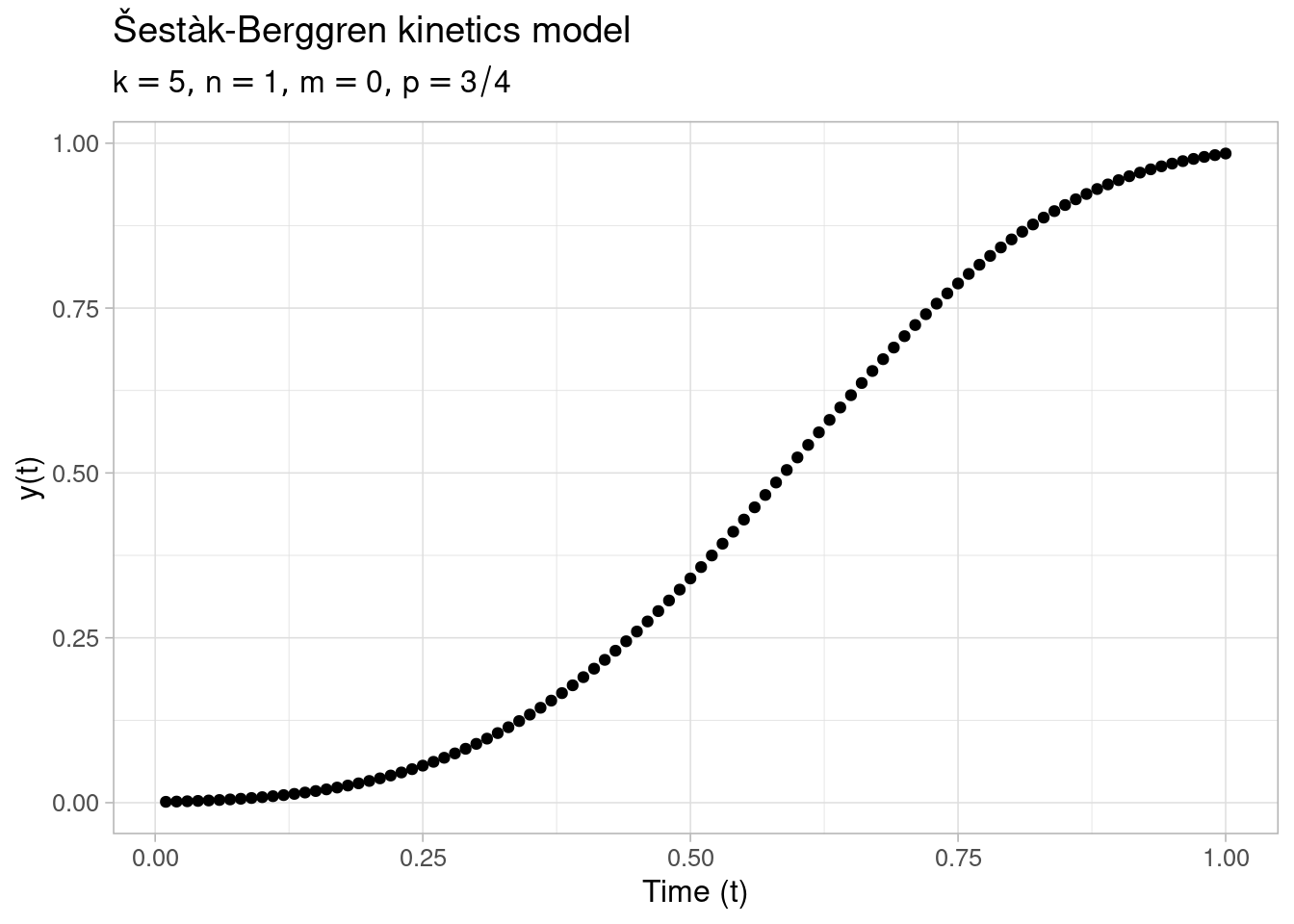### GSL nonlinear least squares fitting in R

October 12, 2021 |

Introduction The new gslnls-package provides R bindings to nonlinear least-squares optimization with the GNU Scientific Library (GSL) using the trust region methods implemented by the gsl_multifit_nlinear module. The gsl_multifit_nlinear module was added in GSL version 2.2 (released in August 2016) and the available nonlinear-least squares routines have been ...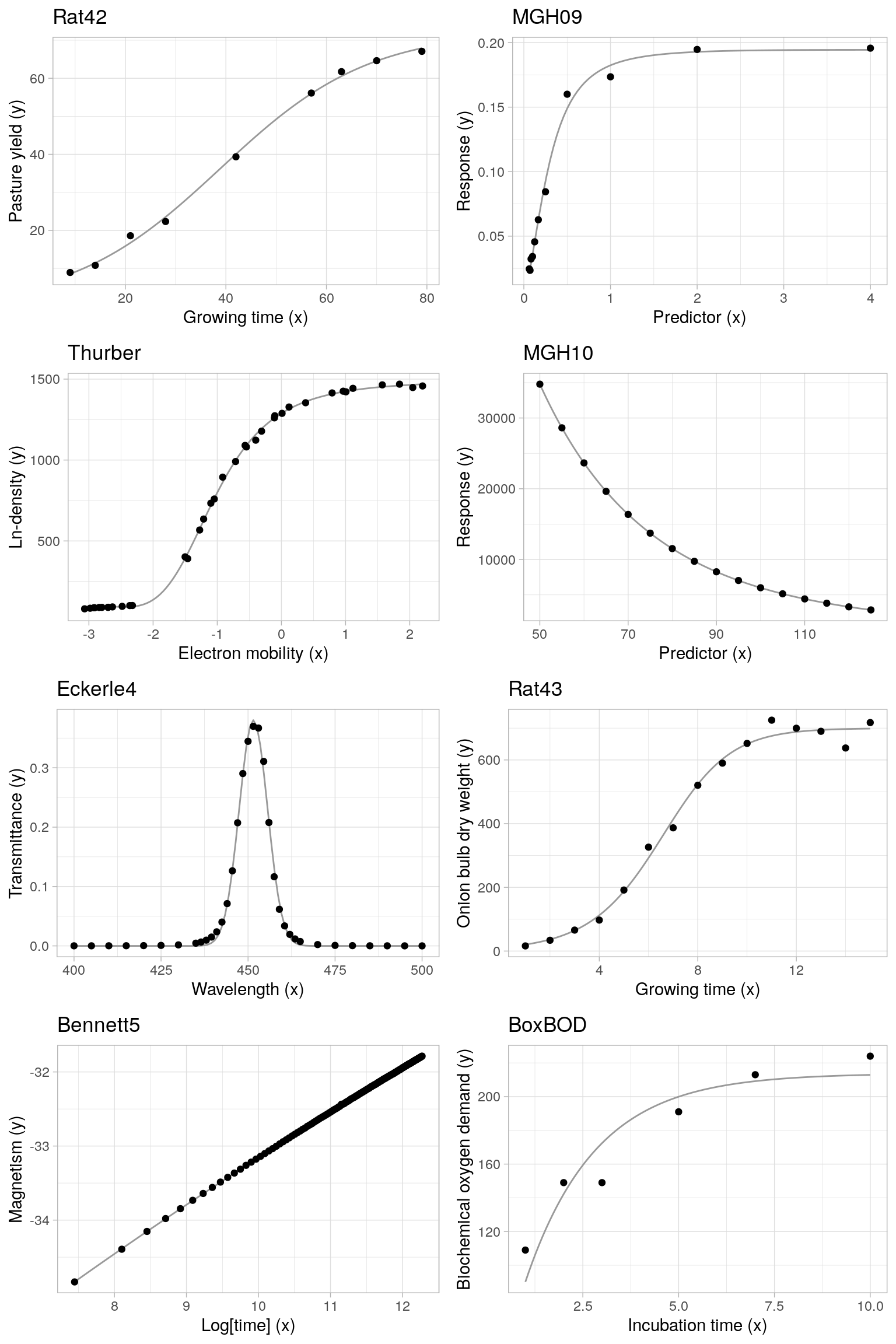July 12, 2021 |

Introduction Nonlinear regression model As a model setup, we consider noisy observations $$y_1,\ldots, y_n \in \mathbb{R}$$ obtained from a standard nonlinear regression model of the form: \begin{aligned} y_i &\ = \ f(\boldsymbol{x}_i, \boldsymbol{\theta}) + \epsilon_i, \quad i = 1,\ldots, n \end{aligned} where $$f: \mathbb{...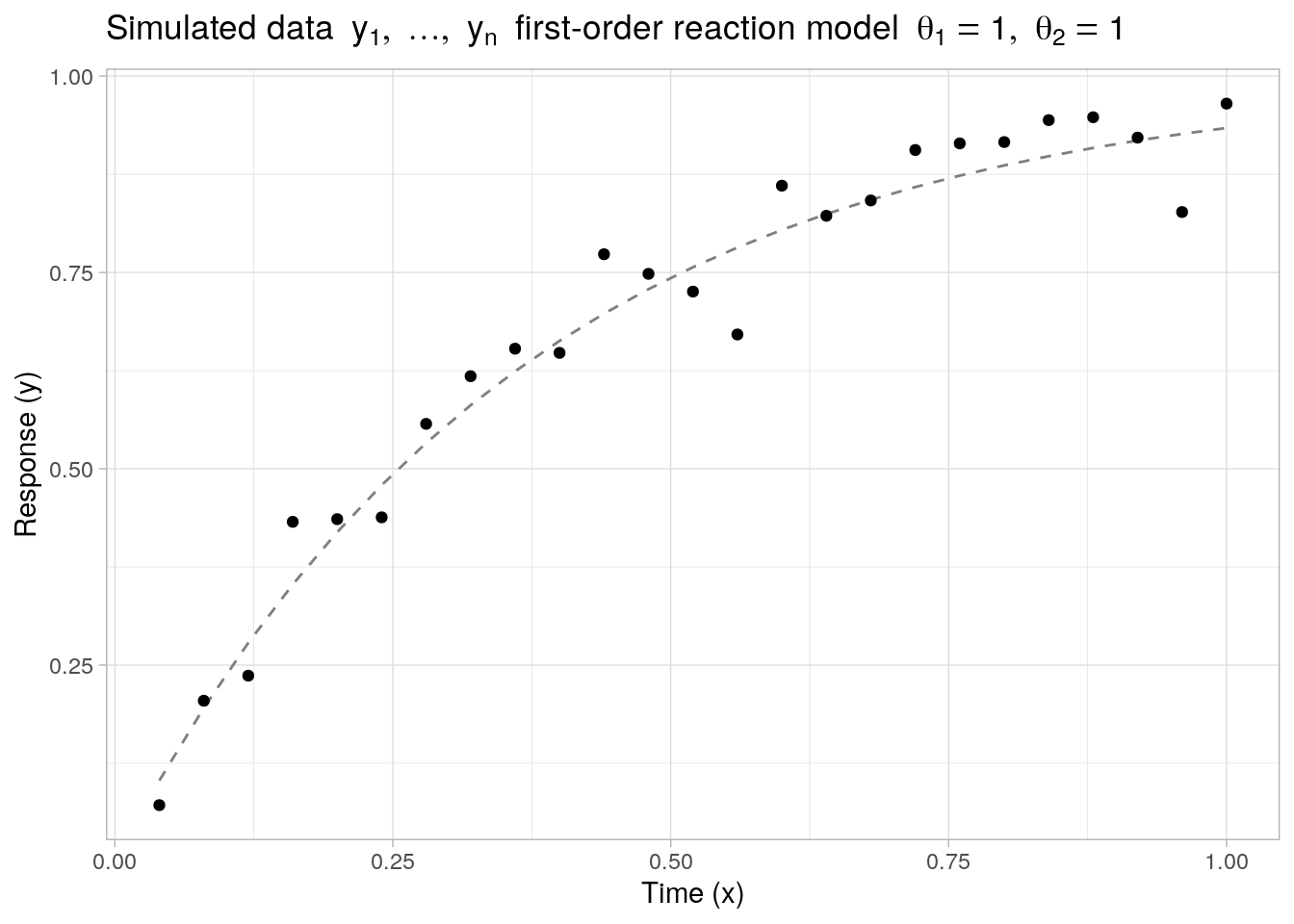[Read more...] ### Step function regression in Stan June 16, 2021 | Introduction Tha aim of this post is to provide a working approach to perform piecewise constant or step function regression in Stan. To set up the regression problem, consider noisy observations \(y_1, \ldots, y_n \in \mathbb{R}$$ sampled from a standard signal plus i.i.d. Gaussian noise model ...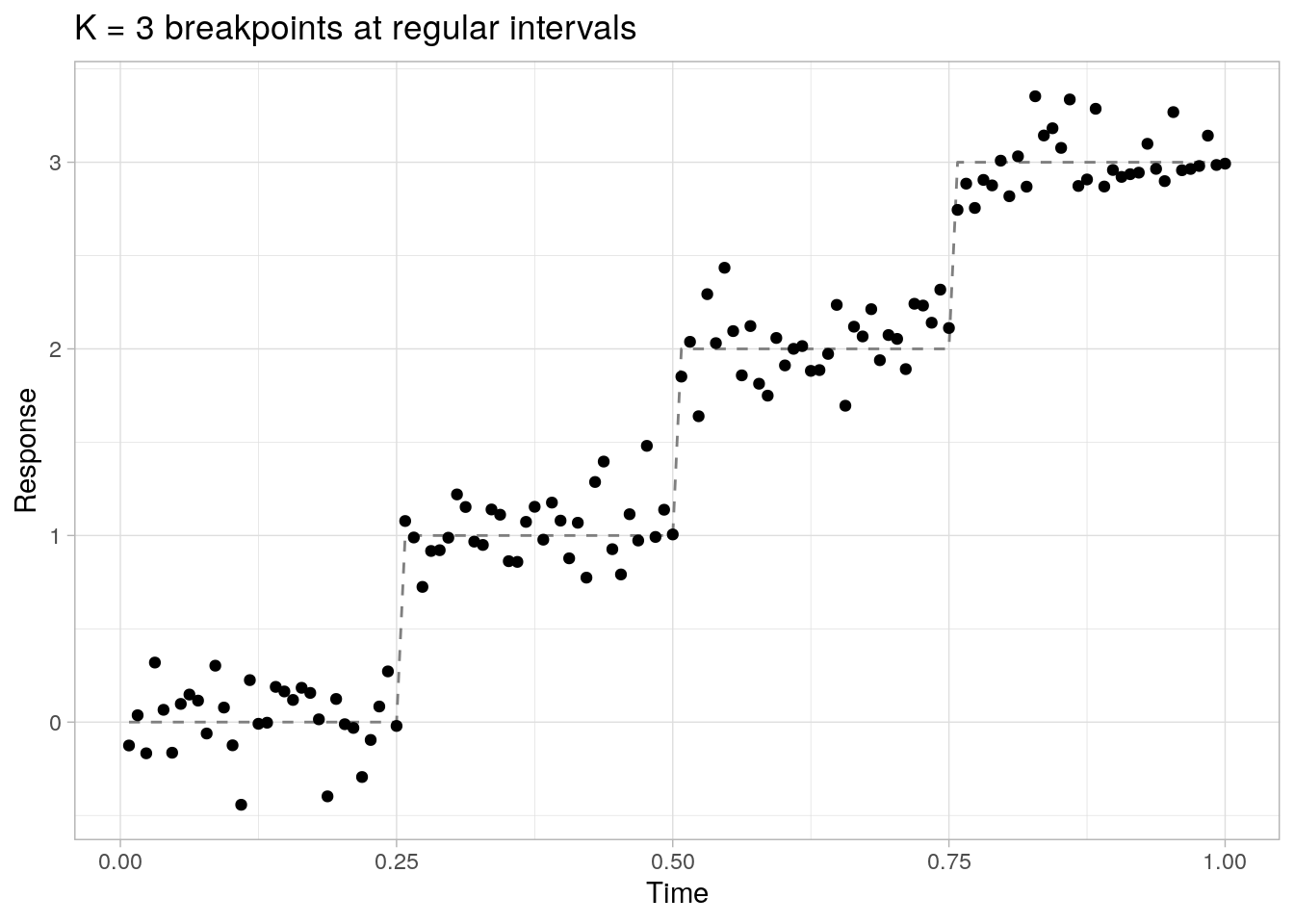### Fitting the Heckman selection model with Stan and R

February 6, 2021 |

Introduction Selection bias Selection bias occurs when sampled data or subjects in a study have been selected in a way that is not representative of the population of interest. As a consequence, conclusions made about the analyzed sample may be difficult to generalize, as the observed effects could be biased ...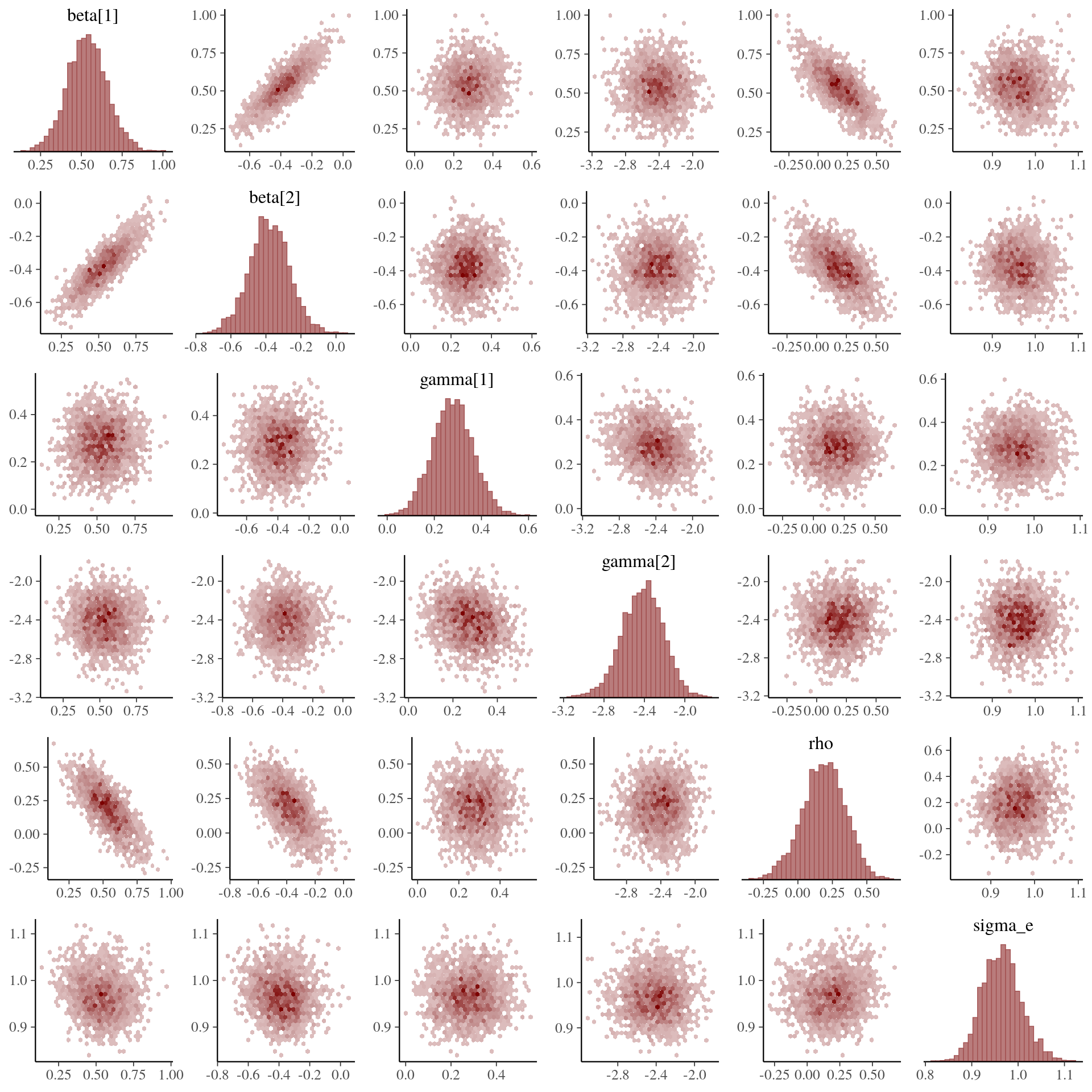### Tracking Stan sampling progress in Shiny

February 1, 2021 |

Introduction The previous post demonstrates the use of pre-compiled Stan models in interactive R Shiny applications to avoid unnecessary Stan model (re-)compilation on application start-up. In this short follow-up post we go a step further and tackle the issue of tracking the Stan model sampling progress itself in a ... [Read more...]

### Running compiled Stan models in Shiny

January 31, 2021 |

Introduction The aim of this post is to provide a short step-by-step guide on writing interactive R Shiny-applications that include models written in Stan using rstan and rstantools. The remainder of this post assumes a small amount of working knowledge on writing models in Stan and usage of the package ... [Read more...]